# KIT phantom dataset tutorial#

Here we read KIT data obtained from a phantom with 49 dipoles sequentially activated with 2-cycle 11 Hz sinusoidal bursts to verify source localization accuracy.

```# Authors: Eric Larson <larson.eric.d@gmail.com>
#
```
```import matplotlib.pyplot as plt
import numpy as np
from scipy.signal import find_peaks

import mne

data_path = mne.datasets.phantom_kit.data_path()
actual_pos, actual_ori = mne.dipole.get_phantom_dipoles("oyama")
actual_pos, actual_ori = actual_pos[:49], actual_ori[:49]  # only 49 of 50 dipoles

# cut from ~800 to ~300s for speed, and also at convenient dip stim boundaries
# chosen by examining MISC 017 by eye.
raw.filter(None, 40)  # 11 Hz stimulation, no need to keep higher freqs
plot_scalings = dict(mag=5e-12)  # large-amplitude sinusoids
raw_plot_kwargs = dict(duration=15, n_channels=50, scalings=plot_scalings)
raw.plot(**raw_plot_kwargs)
```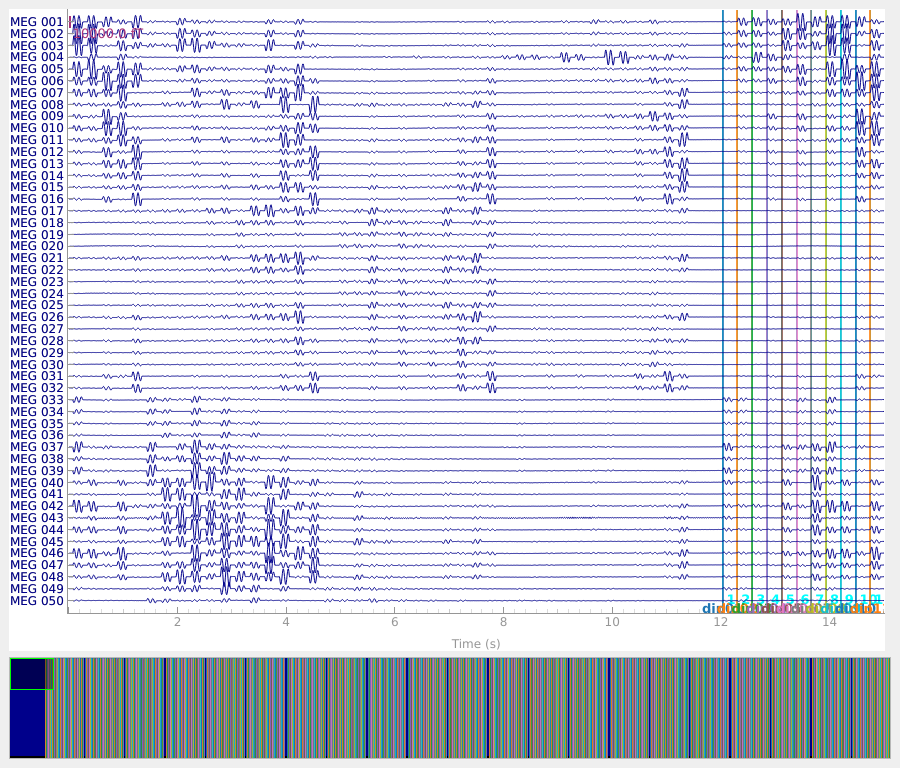```Extracting SQD Parameters from /home/circleci/mne_data/MNE-phantom-KIT-data/002_phantom_11Hz_100uA.con...
Creating Raw.info structure...
Setting channel info structure...
Creating Info structure...
Reading 0 ... 582800  =      0.000 ...   291.400 secs...
Filtering raw data in 1 contiguous segment
Setting up low-pass filter at 40 Hz

FIR filter parameters
---------------------
Designing a one-pass, zero-phase, non-causal lowpass filter:
- Windowed time-domain design (firwin) method
- Hamming window with 0.0194 passband ripple and 53 dB stopband attenuation
- Upper passband edge: 40.00 Hz
- Upper transition bandwidth: 10.00 Hz (-6 dB cutoff frequency: 45.00 Hz)
- Filter length: 661 samples (0.331 s)

[Parallel(n_jobs=1)]: Done  17 tasks      | elapsed:    0.4s
[Parallel(n_jobs=1)]: Done  71 tasks      | elapsed:    1.5s
```

We can also look at the power spectral density to see the phantom oscillations at 11 Hz plus the expected frequency-domain sinc-like oscillations due to the time-domain boxcar windowing of the 11 Hz sinusoid.

```spectrum = raw.copy().crop(0, 60).compute_psd(n_fft=10000)
fig = spectrum.plot()
fig.axes.set_xlim(0, 50)
dip_freq = 11.0
fig.axes.axvline(dip_freq, color="r", ls="--", lw=2, zorder=4)
```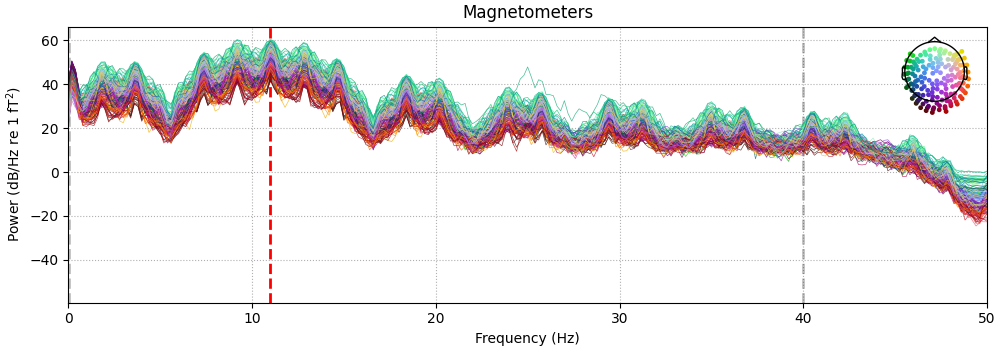```Effective window size : 5.000 (s)
```

To find the events, we can look at the MISC channel that recorded the activations. Here we use a very simple thresholding approach to find the events. The MISC 017 channel holds the dipole activations, which are 2-cycle 11 Hz sinusoidal bursts with the initial sinusoidal deflection downward, so we do a little bit of signal manipulation to help `find_peaks()`.

```# Figure out events
dip_act, dip_t = raw["MISC 017"]
dip_act = dip_act  # 2D to 1D array
dip_act -= dip_act.mean()  # remove DC offset
dip_act *= -1  # invert so first deflection is positive
thresh = np.percentile(dip_act, 90)
min_dist = raw.info["sfreq"] / dip_freq * 0.9  # 90% of period, to be safe
peaks = find_peaks(dip_act, height=thresh, distance=min_dist)
assert len(peaks) % 2 == 0  # 2-cycle modulations
peaks = peaks[::2]  # take only first peaks of each 2-cycle burst

fig, ax = plt.subplots(layout="constrained", figsize=(12, 4))
stop = int(15 * raw.info["sfreq"])  # 15 sec
ax.plot(dip_t[:stop], dip_act[:stop], color="k", lw=1)
ax.axhline(thresh, color="r", ls="--", lw=1)
peak_idx = peaks[peaks < stop]
ax.plot(dip_t[peak_idx], dip_act[peak_idx], "ro", zorder=5, ms=5)
ax.set(xlabel="Time (s)", ylabel="Dipole activation (AU)\n(MISC 017 adjusted)")
ax.set(xlim=dip_t[[0, stop - 1]])

# We know that there are 32 dipoles, so mark the first ones as well
n_dip = 49
assert len(peaks) % n_dip == 0  # we found them all (hopefully)
ax.plot(dip_t[peak_idx[::n_dip]], dip_act[peak_idx[::n_dip]], "bo", zorder=4, ms=10)

# Knowing we've caught the top of the first cycle of a 11 Hz sinusoid, plot onsets
# with red X's.
onsets = peaks - np.round(raw.info["sfreq"] / dip_freq / 4.0).astype(
int
)  # shift to start
onset_idx = onsets[onsets < stop]
ax.plot(dip_t[onset_idx], dip_act[onset_idx], "rx", zorder=5, ms=5)
```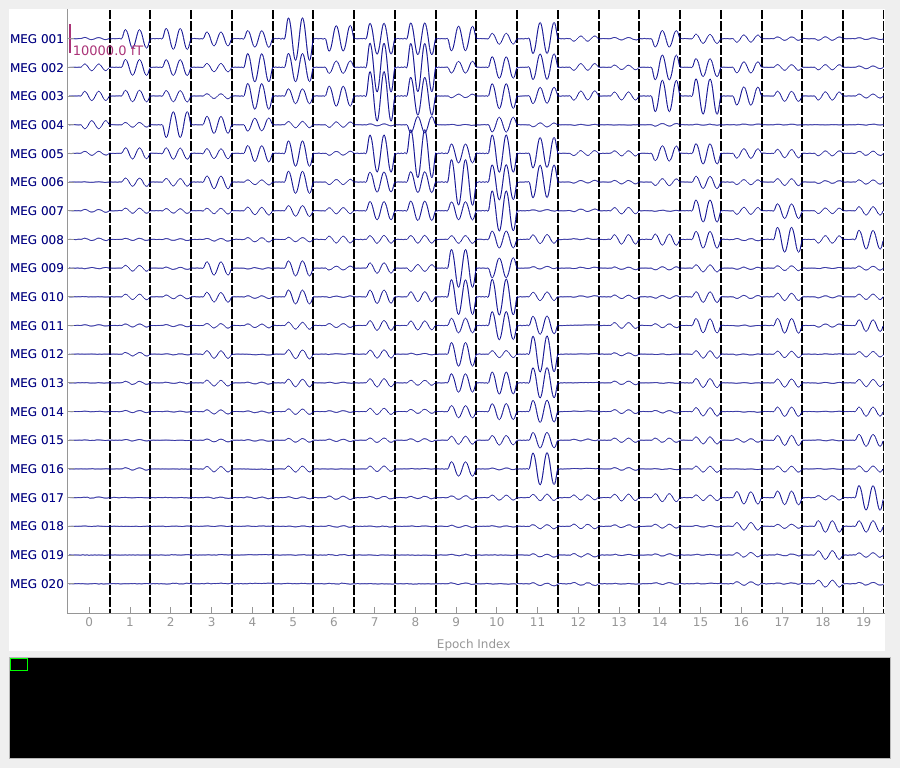Given the onsets are now stored in `peaks`, we can create our events array and plot on our raw data.

```n_rep = len(peaks) // n_dip
events = np.zeros((len(peaks), 3), int)
events[:, 0] = onsets + raw.first_samp
events[:, 2] = np.tile(np.arange(1, n_dip + 1), n_rep)
raw.plot(events=events, **raw_plot_kwargs)
```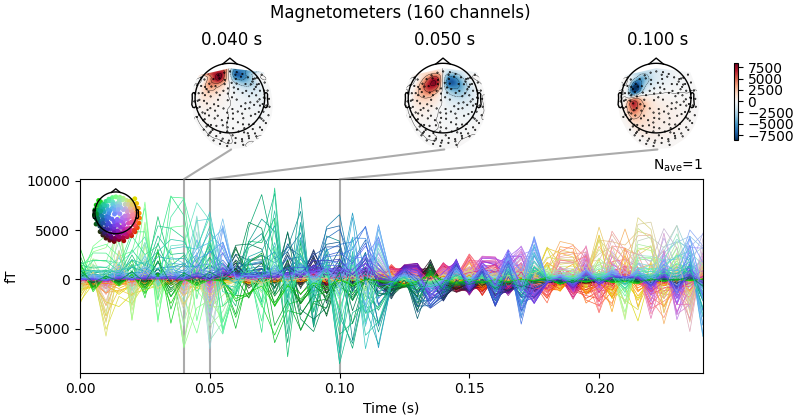Now we can figure out our epoching parameters and epoch the data, sanity checking some values along the way knowing how the stimulation was done.

```# Sanity check and determine epoching params
deltas = np.diff(events[:, 0], axis=0)
group_deltas = deltas[n_dip - 1 :: n_dip] / raw.info["sfreq"]  # gap between 49 and 1
assert (group_deltas > 0.8).all()
assert (group_deltas < 0.9).all()
others = np.delete(deltas, np.arange(n_dip - 1, len(deltas), n_dip))  # remove 49->1
others = others / raw.info["sfreq"]
assert (others > 0.25).all()
assert (others < 0.3).all()
tmax = 1 / dip_freq * 2.0  # 2 cycles
tmin = tmax - others.min()
assert tmin < 0
epochs = mne.Epochs(
raw,
events,
tmin=tmin,
tmax=tmax,
baseline=(None, 0),
decim=10,
picks="data",
)
del raw
epochs.plot(scalings=plot_scalings)
```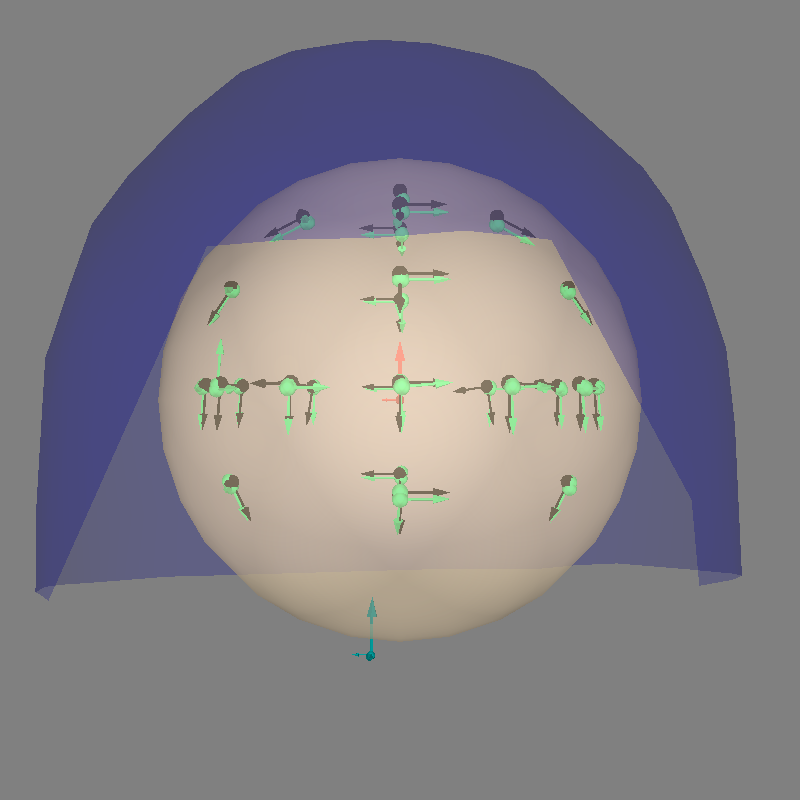```Not setting metadata
1029 matching events found
Setting baseline interval to [-0.085, 0.0] s
Applying baseline correction (mode: mean)
0 projection items activated
Using data from preloaded Raw for 1029 events and 544 original time points (prior to decimation) ...
```

Now we can average the epochs for each dipole, get the activation at the peak time, and create an `mne.EvokedArray` from the result.

```t_peak = 1.0 / dip_freq / 4.0
data = np.zeros((len(epochs.ch_names), n_dip))
for di in range(n_dip):
data[:, [di]] = epochs[str(di + 1)].average().crop(t_peak, t_peak).data
evoked = mne.EvokedArray(data, epochs.info, tmin=0, comment="KIT phantom activations")
evoked.plot_joint()
``````No projector specified for this dataset. Please consider the method self.add_proj.
```

Let’s fit dipoles at each dipole’s peak activation time.

```trans = mne.transforms.Transform("head", "mri", np.eye(4))
cov = mne.compute_covariance(epochs, tmax=0, method="empirical")
# We need to correct the ``dev_head_t`` because it's incorrect for these data!
# relative to the helmet: hleft, forward, up
translation = mne.transforms.translation(x=0.01, y=-0.015, z=-0.088)
rotation = mne.transforms.rotation(
)
dip, residual = mne.fit_dipole(evoked, cov, sphere, n_jobs=None)
```
```Equiv. model fitting -> RV = 0.00364477 %
mu1 = 0.944121    lambda1 = 0.138646
mu2 = 0.665982    lambda2 = 0.684475
mu3 = -0.140083    lambda3 = -0.0127517
Set up EEG sphere model with scalp radius    80.0 mm

Computing rank from data with rank=None
Using tolerance 2.4e-09 (2.2e-16 eps * 160 dim * 6.6e+04  max singular value)
Estimated rank (mag): 160
MAG: rank 160 computed from 160 data channels with 0 projectors
Reducing data rank from 160 -> 160
Estimating covariance using EMPIRICAL
Done.
Number of samples used : 18522
[done]
BEM               : <ConductorModel | Sphere (3 layers): r0=[0.0, 0.0, 0.0] R=80 mm>
MRI transform     : identity
Sphere model      : origin at (   0.00    0.00    0.00) mm, rad =    0.1 mm
Guess grid        :   20.0 mm
Guess mindist     :    5.0 mm
Guess exclude     :   20.0 mm
Using normal MEG coil definitions.
Noise covariance  : <Covariance | kind : full, shape : (160, 160), range : [-1e-26, +2.5e-26], n_samples : 18521>

Coordinate transformation: MRI (surface RAS) -> head
1.000000  0.000000  0.000000       0.00 mm
0.000000  1.000000  0.000000       0.00 mm
0.000000  0.000000  1.000000       0.00 mm
0.000000  0.000000  0.000000       1.00
Coordinate transformation: MEG device -> head
0.998477  0.050618 -0.021924      10.00 mm
-0.052328  0.994909 -0.086126     -15.00 mm
0.017452  0.087142  0.996043     -88.00 mm
0.000000  0.000000  0.000000       1.00
Read 160 MEG channels from info
Coordinate transformation: MEG device -> head
0.998477  0.050618 -0.021924      10.00 mm
-0.052328  0.994909 -0.086126     -15.00 mm
0.017452  0.087142  0.996043     -88.00 mm
0.000000  0.000000  0.000000       1.00
MEG coil definitions created in head coordinates.
Decomposing the sensor noise covariance matrix...
Computing rank from covariance with rank=None
Using tolerance 8.5e-15 (2.2e-16 eps * 160 dim * 0.24  max singular value)
Estimated rank (mag): 160
MAG: rank 160 computed from 160 data channels with 0 projectors
Setting small MAG eigenvalues to zero (without PCA)
Created the whitener using a noise covariance matrix with rank 160 (0 small eigenvalues omitted)

---- Computing the forward solution for the guesses...
Making a spherical guess space with radius    72.0 mm...
Filtering (grid =     20 mm)...
Surface CM = (   0.0    0.0    0.0) mm
Surface fits inside a sphere with radius   72.0 mm
Surface extent:
x =  -72.0 ...   72.0 mm
y =  -72.0 ...   72.0 mm
z =  -72.0 ...   72.0 mm
Grid extent:
x =  -80.0 ...   80.0 mm
y =  -80.0 ...   80.0 mm
z =  -80.0 ...   80.0 mm
729 sources before omitting any.
178 sources after omitting infeasible sources not within 20.0 - 72.0 mm.
170 sources remaining after excluding the sources outside the surface and less than    5.0 mm inside.
Go through all guess source locations...
[done 170 sources]
---- Fitted :     0.0 ms
---- Fitted :     5.0 ms
---- Fitted :    10.0 ms
---- Fitted :    15.0 ms
---- Fitted :    20.0 ms
---- Fitted :    25.0 ms
---- Fitted :    30.0 ms
---- Fitted :    35.0 ms
---- Fitted :    40.0 ms
---- Fitted :    45.0 ms
---- Fitted :    50.0 ms
---- Fitted :    55.0 ms
---- Fitted :    60.0 ms
---- Fitted :    65.0 ms
---- Fitted :    70.0 ms
---- Fitted :    75.0 ms
---- Fitted :    80.0 ms
[Parallel(n_jobs=1)]: Done  17 tasks      | elapsed:    0.9s
---- Fitted :    85.0 ms
---- Fitted :    90.0 ms
---- Fitted :    95.0 ms
---- Fitted :   100.0 ms
---- Fitted :   105.0 ms
---- Fitted :   110.0 ms
---- Fitted :   115.0 ms
---- Fitted :   120.0 ms
---- Fitted :   125.0 ms
---- Fitted :   130.0 ms
---- Fitted :   135.0 ms
---- Fitted :   140.0 ms
---- Fitted :   145.0 ms
---- Fitted :   150.0 ms
---- Fitted :   155.0 ms
---- Fitted :   160.0 ms
---- Fitted :   165.0 ms
---- Fitted :   170.0 ms
---- Fitted :   175.0 ms
---- Fitted :   180.0 ms
---- Fitted :   185.0 ms
---- Fitted :   190.0 ms
---- Fitted :   195.0 ms
---- Fitted :   200.0 ms
---- Fitted :   205.0 ms
---- Fitted :   210.0 ms
---- Fitted :   215.0 ms
---- Fitted :   220.0 ms
---- Fitted :   225.0 ms
---- Fitted :   230.0 ms
---- Fitted :   235.0 ms
---- Fitted :   240.0 ms
49 time points fitted
```

Finally let’s look at the results.

```print(f"Average amplitude: {np.mean(dip.amplitude) * 1e9:0.1f} nAm")
print(f"Average GOF:       {np.mean(dip.gof):0.1f}%")
diffs = 1000 * np.sqrt(np.sum((dip.pos - actual_pos) ** 2, axis=-1))
print(f"Average loc error: {np.mean(diffs):0.1f} mm")
angles = np.rad2deg(np.arccos(np.abs(np.sum(dip.ori * actual_ori, axis=1))))
print(f"Average ori error: {np.mean(angles):0.1f}°")

fig = mne.viz.plot_alignment(
evoked.info,
trans,
bem=sphere,
meg="helmet",
show_axes=True,
)
fig = mne.viz.plot_dipole_locations(
dipoles=dip, mode="arrow", color=(0.2, 1.0, 0.5), fig=fig
)

actual_amp = np.ones(len(dip))  # misc amp to create Dipole instance
actual_gof = np.ones(len(dip))  # misc GOF to create Dipole instance
dip_true = mne.Dipole(dip.times, actual_pos, actual_amp, actual_ori, actual_gof)
fig = mne.viz.plot_dipole_locations(
dipoles=dip_true, mode="arrow", color=(0.0, 0.0, 0.0), fig=fig
)

mne.viz.set_3d_view(figure=fig, azimuth=90, elevation=90, distance=0.5)
``````Average amplitude: 578.7 nAm
Average GOF:       99.6%
Average loc error: 1.7 mm
Average ori error: 9.6°
Getting helmet for system KIT (derived from 160 MEG channel locations)
```

Total running time of the script: (0 minutes 46.178 seconds)

Estimated memory usage: 2083 MB

Gallery generated by Sphinx-Gallery